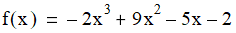# NMath User's Guide

33.2 Finding Function Roots of Derivable Functions (.NET, C#, CSharp, VB, Visual Basic, F#)

Class NewtonRalphsonRootFinder implements the interface and finds roots of univariate functions using the Newton-Raphson Method. The Newton-Raphson algorithm finds the slope of the function at the current point and uses the zero of the tangent line as an estimate of the root.

Like SecantRootFinder and RiddersRootFinder (Section 33.1), instances of NewtonRalphsonRootFinder are constructed by specifying an error tolerance and a maximum number of iterations, or by accepting the defaults for these values. For example:

Code Example – C# root finding

```double tol = 1e-8;
```
```int maxIter = 100;
```
```var finder = new NewtonRaphsonRootFinder( tol, maxIter );
```

Code Example – VB root finding

```Dim Tol As Double = "1e-8"
```
```Dim MaxIter As Integer = 100
```
```Dim Finder As New NewtonRaphsonRootFinder(Tol, MaxIter)
```

Once you have constructed a NewtonRalphsonRootFinder instance, you can use the Find() method to find a root within a given interval. For instance, this polynomial has a root at 1:This code finds the root in the interval (0, 3):

Code Example – C# root finding

```var p = new Polynomial(
```
```  new DoubleVector( -2.0, -5.0, 9.0, -2.0 ) );
```
```var finder = new NewtonRaphsonRootFinder();
```
```double lower = 0;
```
```double upper = 3;
```
```double root = finder.Find( p, p.Derivative(), lower, upper );
```

Code Example – VB root finding

```Dim P As New Polynomial(New DoubleVector(-2.0, -5.0, 9.0, -2.0))
```
```Dim Finder As New NewtonRaphsonRootFinder()
```
```Dim Lower As Double = 0
```
```Dim Upper As Double = 3
```
```Dim Root As Double = Finder.Find(P, P.Derivative(), Lower, Upper)

```

Top

Top